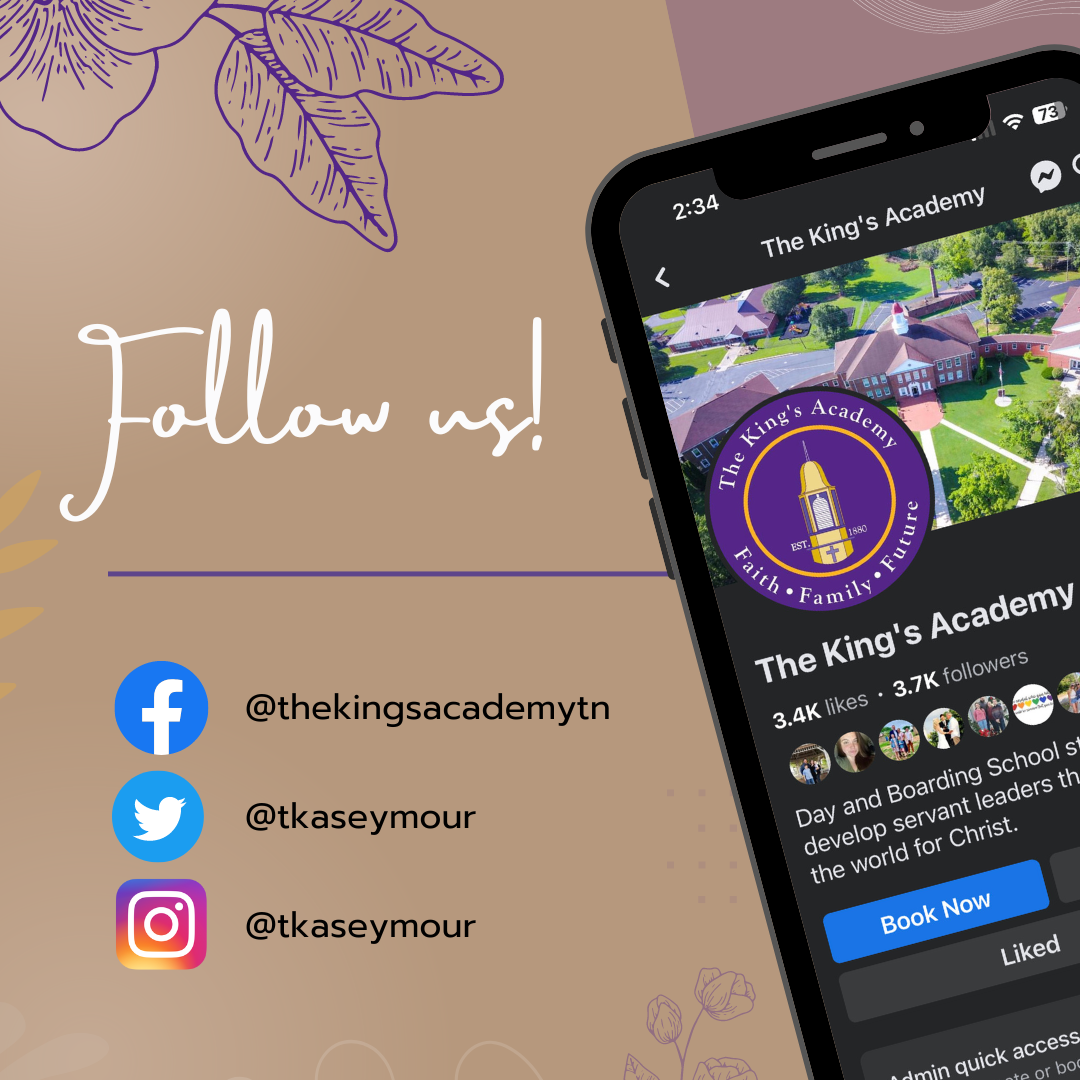## Lions Weekly: August 16, 2023# A Note From Mr. Sandefur

Lion Family,

I hope this email finds you well after our first full week back at school. I want to express my heartfelt gratitude for your flexibility and understanding as we navigated through the weather challenges that marked the beginning of the academic year. Your support and partnership in creating a positive learning environment for our students are truly appreciated.

In Hebrews 13:21, we find a profound reminder that speaks to our core values at The King's Academy. As we work together to equip our students with every good thing for doing God's will, may we be reminded that the challenges we face often contribute to the growth and development of our children. Just as a blacksmith tempers and shapes iron through fire, the difficulties we encounter play a part in forming the character of our students. Our portrait of a graduate emphasizes the importance of being "equipped" not only academically but also spiritually and morally, guiding our students to become well-rounded individuals who are ready to make a positive impact on the world.

As we move forward into the new week, let's continue to foster a spirit of resilience, adaptability, and a firm grounding in faith. Your partnership is integral to the success of our mission, and I am excited to see the growth and achievements that lie ahead for each student. Thank you for entrusting us with your child's education and character development.

In Christ,

Jeremy Sandefur

 table div table+table+table+table div table{width:100%;padding:0}table div table+table+table+table div table img{width:96.23%;padding:0;float:none}table div table+table+table+table div table td{width:100%;padding:0 1.88% 18px}/* styles */table div table+table+table+table+table+table div table{width:100%;padding:0}table div table+table+table+table+table+table div table img{width:96.23%;padding:0;float:none}table div table+table+table+table+table+table div table td{width:100%;padding:0 1.88% 18px}/* styles */# Grandparents Day

Mark your calendars! Grandparents Day is just around the corner on September. Stay tuned for more details from Mrs. Sullivan. It's a wonderful opportunity to celebrate and cherish the bond between generations.

 table div table+table+table+table+table+table+table+table div table{width:100%;padding:0}table div table+table+table+table+table+table+table+table div table img{width:96.23%;padding:0;float:none}table div table+table+table+table+table+table+table+table div table td{width:100%;padding:0 1.88% 18px}/* styles */# Host Family Update

TKA Family! As our Residence Life program continues to grow, we are still looking for two host families to join us! Being a host family for our Residence Life students doesn't entail shouldering their physical or financial needs. We understand that there might be misconceptions about the extent of this commitment. While many host families go above and beyond, forming deep bonds with the students, we want to emphasize that this isn't the expectation. We encourage you to step out in faith, embrace a new family member, and positively impact their future.
-Tasha

 table div table+table+table+table+table+table+table+table+table+table+table div table{width:100%;padding:0}table div table+table+table+table+table+table+table+table+table+table+table div table img{width:96.23%;padding:0;float:none}table div table+table+table+table+table+table+table+table+table+table+table div table td{width:100%;padding:0 1.88% 18px}/* styles */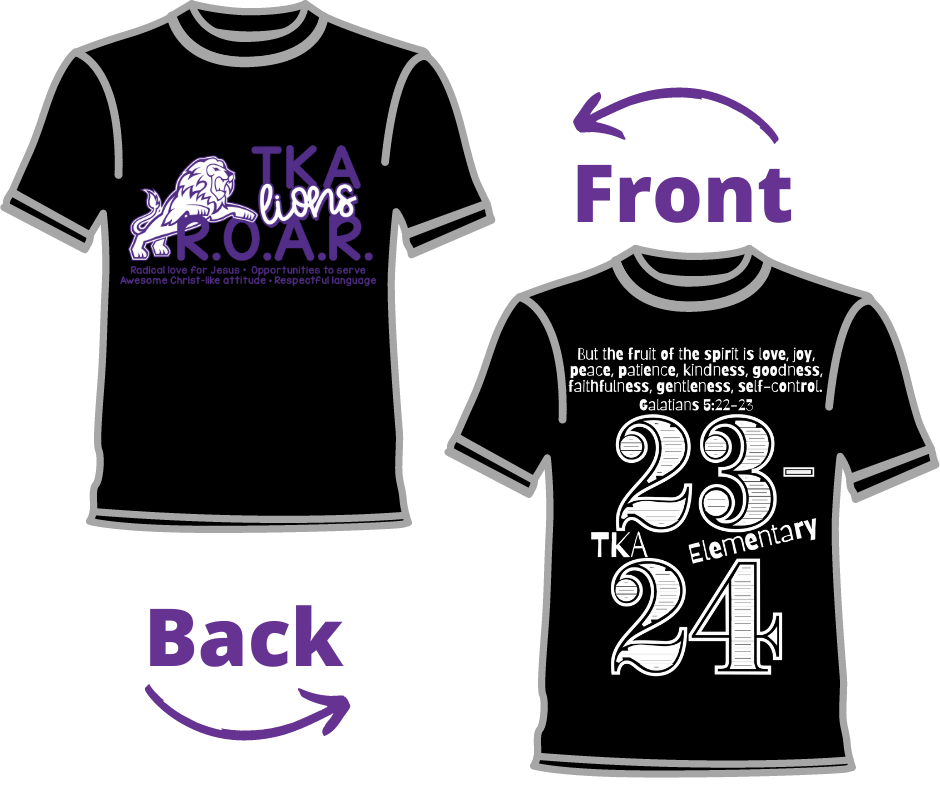# R.O.A.R. Shirt Order Forms!

We hope your child is settling in well for the new school year. As part of our school spirit and identity, we are excited to offer Elementary Shirts for our students. These shirts not only create a sense of unity among our students but also allow them to proudly represent The King's Academy on campus, in the community, and on field trips. These shirts are free for students, however, we also have the option for family and friends to purchase as well. We kindly encourage you to fill out the order form and submit the payment by Friday, August 25th. Your timely response will help us ensure that every student receives their shirt promptly. Thank you for your support!

 table div table+table+table+table+table+table+table+table+table+table+table+table+table+table+table div table{width:100%;padding:0}table div table+table+table+table+table+table+table+table+table+table+table+table+table+table+table div table img{width:96.23%;padding:0;float:none}table div table+table+table+table+table+table+table+table+table+table+table+table+table+table+table div table td{width:100%;padding:0 1.88% 18px}/* styles */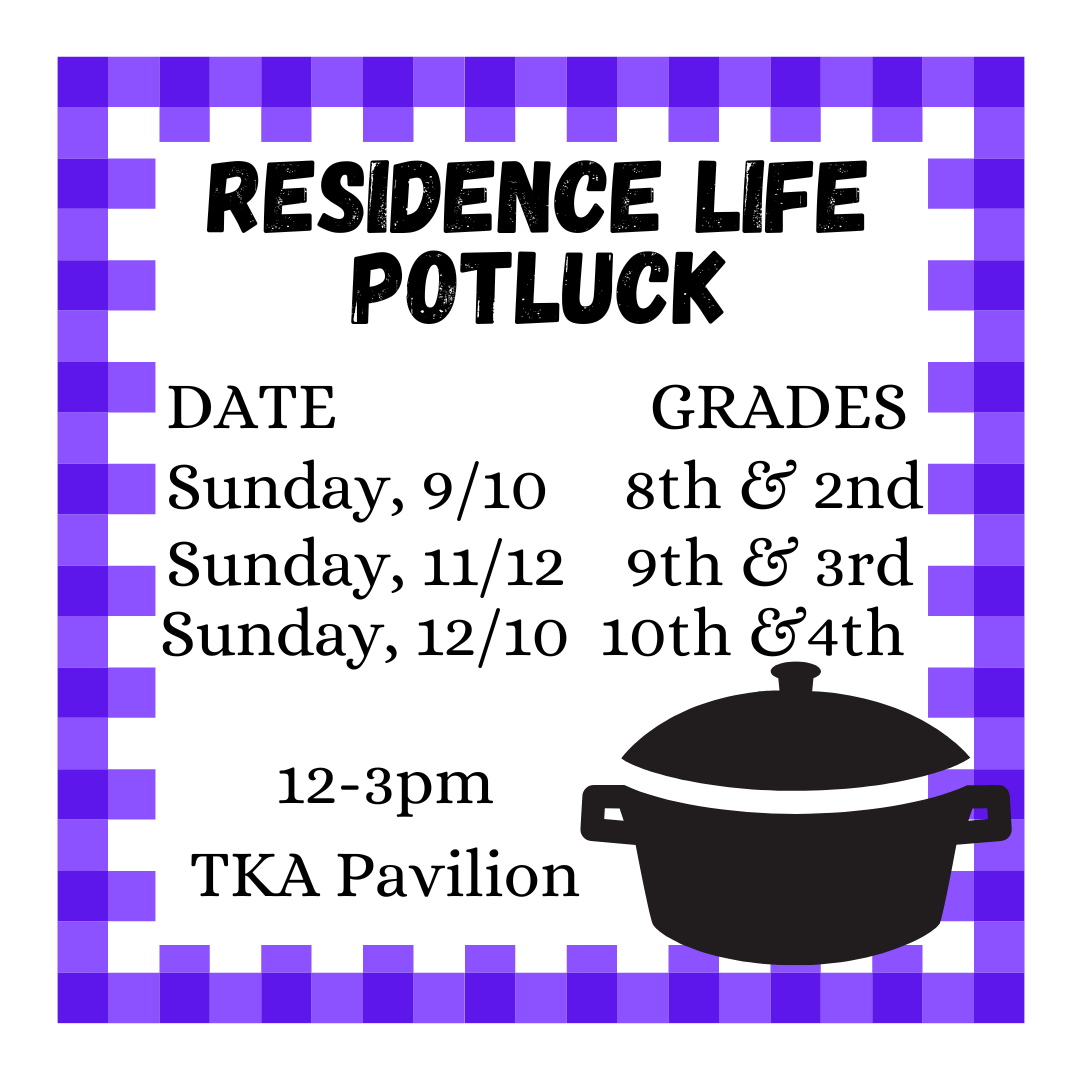# Residence Life Potluck

This past Sunday was our first Residence Life Potluck. PTF came alongside Residence Life to give our international students a taste of American culture and allow a chance for students and families to get to know new people. Residence Life will continue to host one potluck a month, inviting families from different grades. Our vision is to bring people within our TKA family together, whose paths may not cross otherwise. Stay tuned for more information in the near future!- Tasha Wehrle

 table div table+table+table+table+table+table+table+table+table+table+table+table+table+table+table+table+table div table{width:100%;padding:0}table div table+table+table+table+table+table+table+table+table+table+table+table+table+table+table+table+table div table img{width:96.23%;padding:0;float:none}table div table+table+table+table+table+table+table+table+table+table+table+table+table+table+table+table+table div table td{width:100%;padding:0 1.88% 18px}/* styles */The school board met this week and made revisions to this year's student handbook. Be sure to review the updated handbook below! Your partnership is valued as we work together for a successful school year.

 table div table+table+table+table+table+table+table+table+table+table+table+table+table+table+table+table+table+table+table+table div table{width:100%;padding:0}table div table+table+table+table+table+table+table+table+table+table+table+table+table+table+table+table+table+table+table+table div table img{width:96.23%;padding:0;float:none}table div table+table+table+table+table+table+table+table+table+table+table+table+table+table+table+table+table+table+table+table div table td{width:100%;padding:0 1.88% 18px}/* styles */# The Faces And Our Cultures Fall Program

The King's Academy has a wonderful opportunity to participate in a cultural exchange program for Guatemalan students and the optional opportunity to visit Guatemala next summer in a reciprocal way.

The Faces And Our Cultures Fall program is designed to provide Guatemalan students the opportunity to spend 8 weeks in our community.

We are looking for a set of families to host students during weekends and Thanksgiving break. Each participant will attend The King's Academy for eight weeks as boarding students. They will have their own schedule and will participate in all aspects of school life. These student ambassadors are fluent in English and will arrive on Saturday, October 14th, and depart on Saturday, December 9th.

Host families kindly provide homestay and make them part of their family's routine during WEEKENDS for 7 weeks and on our WEEK-LONG Thanksgiving break.

The program goes beyond practicing a second language for Guatemalan and US students. It is an opportunity for them to push their limits, learn firsthand from another culture, and have a life-enriching experience. Finally, students and families create lasting connections that transcend borders.

In order to be considered cultural ambassadors of their country, these participants must apply, go through a rigorous selection process, and have undergone extensive preparation throughout the year.

We will have an in-person informational meeting with Sandy Cardona, our coordinator with Faces on August 28, 2023, @ 3:30pm
-April Kandel

 table div table+table+table+table+table+table+table+table+table+table+table+table+table+table+table+table+table+table+table+table+table+table+table div table{width:100%;padding:0}table div table+table+table+table+table+table+table+table+table+table+table+table+table+table+table+table+table+table+table+table+table+table+table div table img{width:96.23%;padding:0;float:none}table div table+table+table+table+table+table+table+table+table+table+table+table+table+table+table+table+table+table+table+table+table+table+table div table td{width:100%;padding:0 1.88% 18px}/* styles */## Homecoming Information!# TURN UP THE SPIRIT!

Get ready to ignite the school spirit as we announce this year's thrilling Homecoming theme: "Turn Up the Spirit"! Prepare for an unforgettable week of festivities, camaraderie, and celebrating our Lion pride like never before.# Monday: Light-It Up! (September 18)

Get ready to shine on Light-It Up Day with vibrant neon colors! Let's brighten up the campus by wearing your favorite neon clothing as we kick off Homecoming Week in style.

all attire must adhere to dress code standards# Tuesday: Team-Up Day (September 19)

Support your favorite sports team by wearing their colors or jersey on Team-Up Day. Show off your team pride and get into the competitive spirit!

all attire must adhere to dress code standards# Wednesday: Grown-Up Day (September 20)

Embrace your future aspirations on Grown-Up Day! Dress up as the professional you aspire to be, whether it's a doctor, scientist, or any other career.

all attire must adhere to dress code standards# Thursday: Party-Up Day (September 21)

It's time to celebrate on Party-Up Day! Dress in your favorite holiday attire and get in the festive mood.

all attire must adhere to dress code standards# Friday: Squad-Up Day (September 22)

Wear your Homecoming shirt with pride on Squad-Up Day. Available in both purple and black, each grade level has been assigned a color. Let's show our unity and Lion pride as we wrap up this exciting week!

all attire must adhere to dress code standards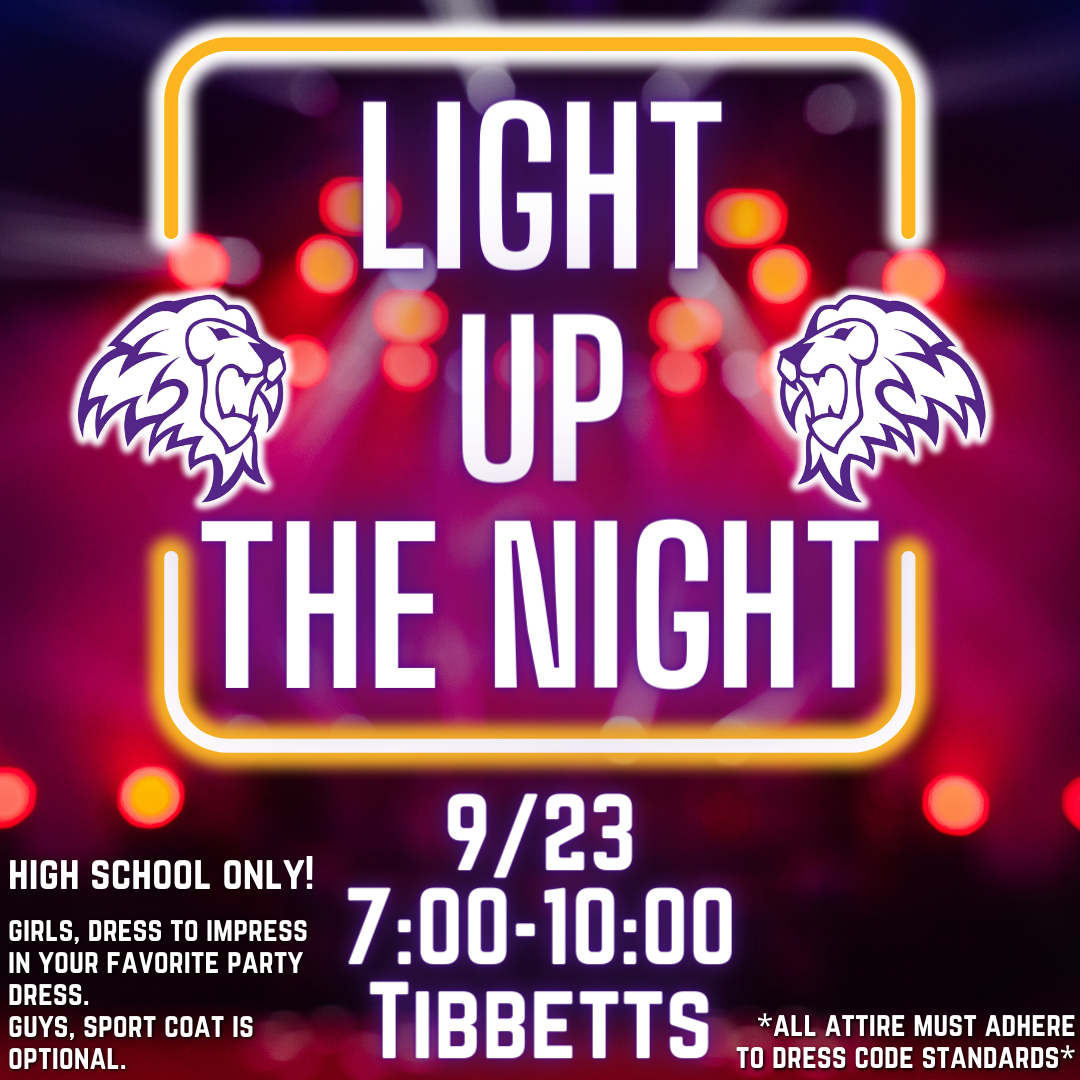# Homecoming Dance: "Light Up the Night"

Get ready to illuminate the night at this year's Homecoming Dance, themed "Light Up the Night"! High school students are invited to join us on September 23 from 7:00 to 10:00 PM for an evening of music, dancing, and unforgettable memories. Make sure to adhere to dress code standards; all attire must be approved, especially dresses. Let's make this a dazzling and memorable Homecoming celebration!

 table div table+table+table+table+table+table+table+table+table+table+table+table+table+table+table+table+table+table+table+table+table+table+table+table+table+table+table+table+table+table+table+table+table+table div table{width:100%;padding:0}table div table+table+table+table+table+table+table+table+table+table+table+table+table+table+table+table+table+table+table+table+table+table+table+table+table+table+table+table+table+table+table+table+table+table div table img{width:96.23%;padding:0;float:none}table div table+table+table+table+table+table+table+table+table+table+table+table+table+table+table+table+table+table+table+table+table+table+table+table+table+table+table+table+table+table+table+table+table+table div table td{width:100%;padding:0 1.88% 18px}/* styles */## Aug. 14-19 Sports Schedule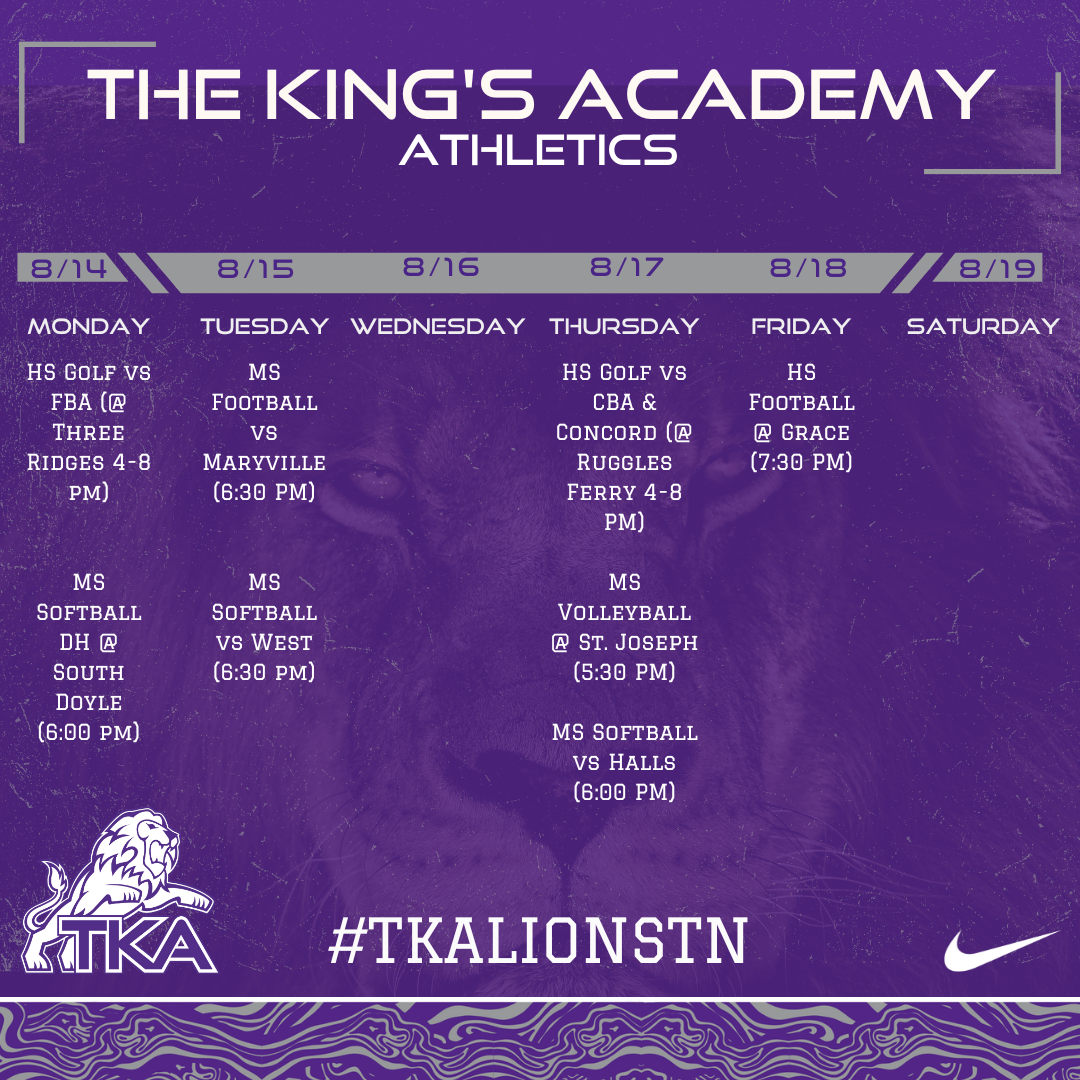table div table+table+table+table+table+table+table+table+table+table+table+table+table+table+table+table+table+table+table+table+table+table+table+table+table+table+table+table+table+table+table+table+table+table+table+table+table div table{width:100%;padding:0}table div table+table+table+table+table+table+table+table+table+table+table+table+table+table+table+table+table+table+table+table+table+table+table+table+table+table+table+table+table+table+table+table+table+table+table+table+table div table img{width:96.23%;padding:0;float:none}table div table+table+table+table+table+table+table+table+table+table+table+table+table+table+table+table+table+table+table+table+table+table+table+table+table+table+table+table+table+table+table+table+table+table+table+table+table div table td{width:100%;padding:0 1.88% 18px}/* styles */## Lunch Menu for Aug. 21-Sept.1table div table+table+table+table+table+table+table+table+table+table+table+table+table+table+table+table+table+table+table+table+table+table+table+table+table+table+table+table+table+table+table+table+table+table+table+table+table+table+table+table+table div table{width:100%;padding:0}table div table+table+table+table+table+table+table+table+table+table+table+table+table+table+table+table+table+table+table+table+table+table+table+table+table+table+table+table+table+table+table+table+table+table+table+table+table+table+table+table+table div table img{width:96.23%;padding:0;float:none}table div table+table+table+table+table+table+table+table+table+table+table+table+table+table+table+table+table+table+table+table+table+table+table+table+table+table+table+table+table+table+table+table+table+table+table+table+table+table+table+table+table div table td{width:100%;padding:0 1.88% 18px}/* styles */# Instructional Calendar

 table div table+table+table+table+table+table+table+table+table+table+table+table+table+table+table+table+table+table+table+table+table+table+table+table+table+table+table+table+table+table+table+table+table+table+table+table+table+table+table+table+table+table+table+table div table td,table.module-43{width:100%;padding:0}table div table+table+table+table+table+table+table+table+table+table+table+table+table+table+table+table+table+table+table+table+table+table+table+table+table+table+table+table+table+table+table+table+table+table+table+table+table+table+table+table+table+table+table+table div table{width:100%;float:none;margin-left:auto;margin-right:auto;padding:0}table div table+table+table+table+table+table+table+table+table+table+table+table+table+table+table+table+table+table+table+table+table+table+table+table+table+table+table+table+table+table+table+table+table+table+table+table+table+table+table+table+table+table+table+table div table a{border:0 none;text-decoration:none}table div table+table+table+table+table+table+table+table+table+table+table+table+table+table+table+table+table+table+table+table+table+table+table+table+table+table+table+table+table+table+table+table+table+table+table+table+table+table+table+table+table+table+table+table div table img{width:100%!important;border:0 none;text-decoration:none}/* styles */

# Dress Code

 table div table+table+table+table+table+table+table+table+table+table+table+table+table+table+table+table+table+table+table+table+table+table+table+table+table+table+table+table+table+table+table+table+table+table+table+table+table+table+table+table+table+table+table+table+table+table div table td,table.module-45{width:100%;padding:0}table div table+table+table+table+table+table+table+table+table+table+table+table+table+table+table+table+table+table+table+table+table+table+table+table+table+table+table+table+table+table+table+table+table+table+table+table+table+table+table+table+table+table+table+table+table+table div table{width:100%;float:none;margin-left:auto;margin-right:auto;padding:0}table div table+table+table+table+table+table+table+table+table+table+table+table+table+table+table+table+table+table+table+table+table+table+table+table+table+table+table+table+table+table+table+table+table+table+table+table+table+table+table+table+table+table+table+table+table+table div table a{border:0 none;text-decoration:none}table div table+table+table+table+table+table+table+table+table+table+table+table+table+table+table+table+table+table+table+table+table+table+table+table+table+table+table+table+table+table+table+table+table+table+table+table+table+table+table+table+table+table+table+table+table+table div table img{width:100%!important;border:0 none;text-decoration:none}/* styles */

# Immunizations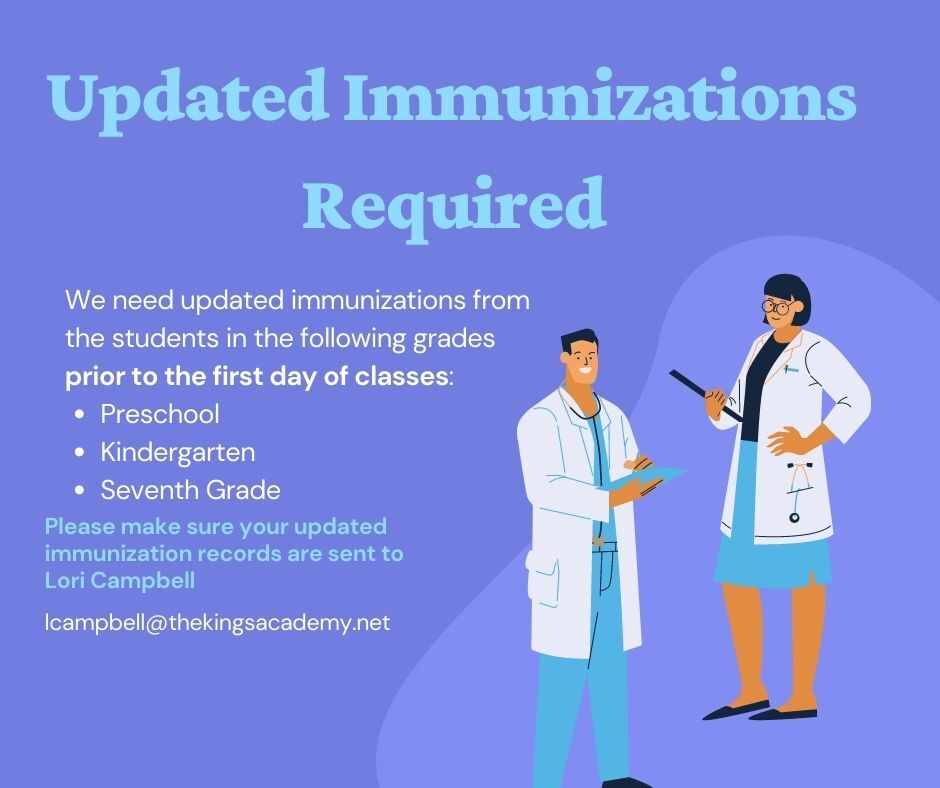table div table+table+table+table+table+table+table+table+table+table+table+table+table+table+table+table+table+table+table+table+table+table+table+table+table+table+table+table+table+table+table+table+table+table+table+table+table+table+table+table+table+table+table+table+table+table+table+table+table+table+table div table{width:100%;padding:0}table div table+table+table+table+table+table+table+table+table+table+table+table+table+table+table+table+table+table+table+table+table+table+table+table+table+table+table+table+table+table+table+table+table+table+table+table+table+table+table+table+table+table+table+table+table+table+table+table+table+table+table div table img{width:96.23%;padding:0;float:none}table div table+table+table+table+table+table+table+table+table+table+table+table+table+table+table+table+table+table+table+table+table+table+table+table+table+table+table+table+table+table+table+table+table+table+table+table+table+table+table+table+table+table+table+table+table+table+table+table+table+table+table div table td{width:100%;padding:0 1.88% 18px}/* styles */## Reminders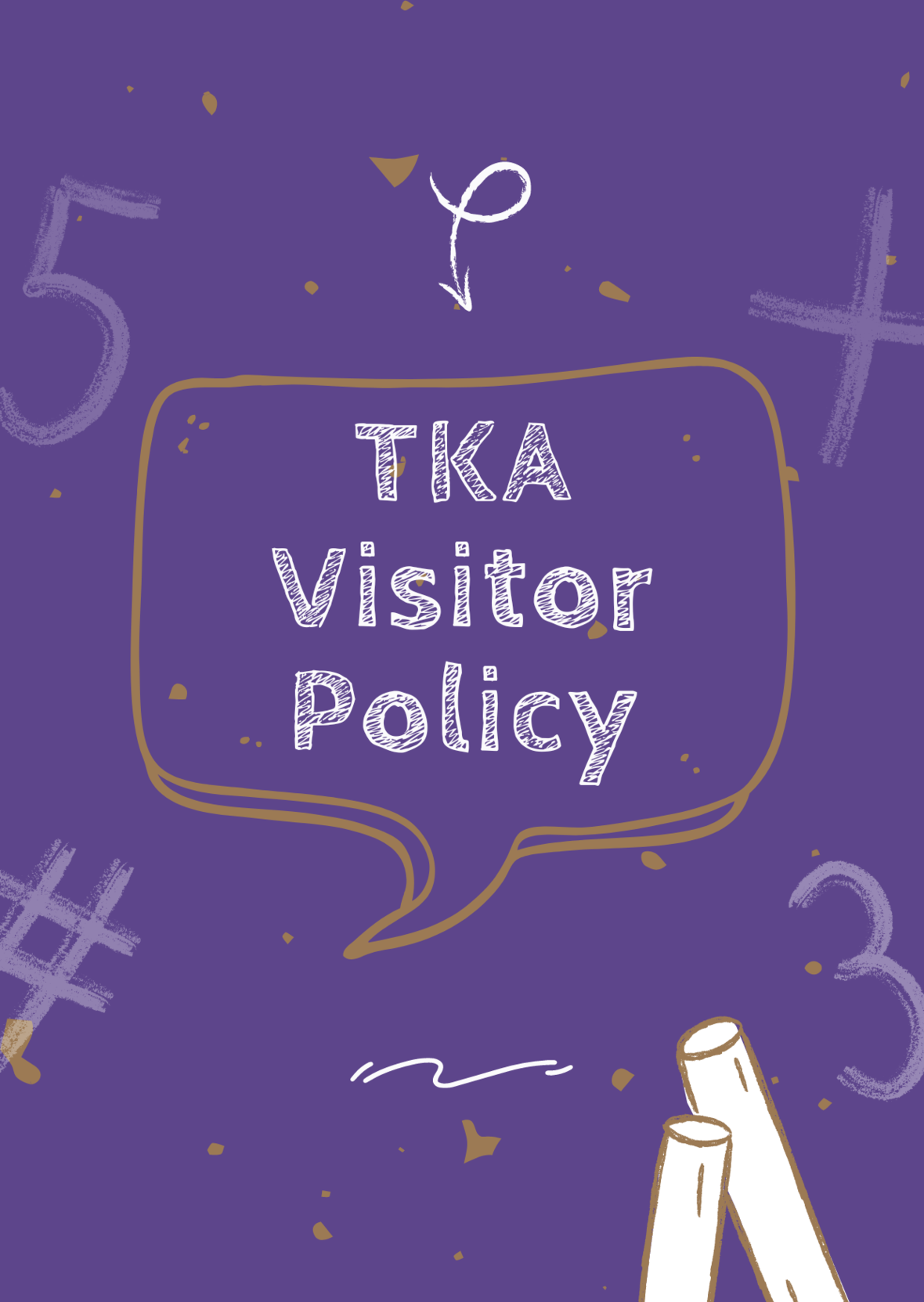# TKA Visitor Policy

In order to help maintain a safe and instructionally based learning environment for our students, all visitors for middle and high school must enter through the front door of the Anderson Building and sign in in the middle/high school office in order to receive a visitor's pass. All visitors for elementary must enter the front door of the Ogle Building in order to receive a visitor's pass. This pass should be visible at all times so that TKA faculty and staff know that you have checked in at the office.

Our doors are open to visitors, but please call ahead of time to schedule conferences with your child’s teachers. This will prevent interruptions to instruction and the loss of the valuable learning time of our students. Adults will not be allowed to wander the halls of the building or hold conferences with teachers during instructional times.

TKA family lunch visitors should follow the above procedure. Lunch tickets are \$5 each.

Students who are not enrolled at TKA are not permitted to visit TKA students during the school day, which includes lunch.

This policy has been developed in order to help maintain a safe and instruction focused environment at school each day. Any visitor who does not meet these expectations may be asked to leave school grounds.

 table div table+table+table+table+table+table+table+table+table+table+table+table+table+table+table+table+table+table+table+table+table+table+table+table+table+table+table+table+table+table+table+table+table+table+table+table+table+table+table+table+table+table+table+table+table+table+table+table+table+table+table+table+table+table div table{width:100%;padding:0}table div table+table+table+table+table+table+table+table+table+table+table+table+table+table+table+table+table+table+table+table+table+table+table+table+table+table+table+table+table+table+table+table+table+table+table+table+table+table+table+table+table+table+table+table+table+table+table+table+table+table+table+table+table+table div table img{width:96.23%;padding:0;float:none}table div table+table+table+table+table+table+table+table+table+table+table+table+table+table+table+table+table+table+table+table+table+table+table+table+table+table+table+table+table+table+table+table+table+table+table+table+table+table+table+table+table+table+table+table+table+table+table+table+table+table+table+table+table+table div table td{width:100%;padding:0 1.88% 18px}/* styles */